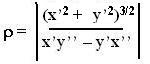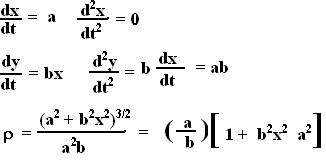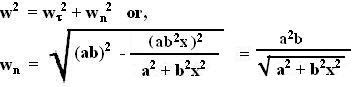## Friday, July 20, 2007

### Irodov Problem 1.35

(a) Actually this is almost identical to the previous problem since,Now we can find the trajectory of the particle as,(b) We can solve this problem both mathematically or using the expression for radial acceleration.

Using Mathematical definition for radius of curvature:
We know that the radius of curvature is given by, here x' and x'' represent the first and second derivatives with respect to time and the same for y.(why? see here for the explanation).

From (1a,b) we have,Using radial acceleration () :
The total acceleration of the particle is given by ab, as seen in above. The tangential acceleration of the particle can be determined by projecting the acceleration vector along the velocity vector to give,The acceleration of the particle in the normal direction can be determined as,The tangential velocity of the particle is same as the magnitude of the velocity vector given by |v|. Thus the radial acceleration is also given by,This implies that,Anonymous said...

in the book answer, the radius of curvature has been given by velocity squared divided by centripetal acceleration. how do we do it from that method?Anonymous said...

in the book answer, the radius of curvature has been given by velocity squared divided by centripetal acceleration. how do we do it from that method?

Krishna Kant Chintalapudi said...

The alternative solution using your definition is also provided - see the part that says "using radial acceleration"